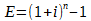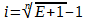The effective interest rate is what makes the calculation come out to truth. You want both arrangements to come out to be the exact same effective rate. So, the effective interest is calculated using the number of compounding interest recalculations. Then the calculation is backed out into the number of payments. That way, both will calculate the same effective interest. E is the effective interest for the year. i is the rate of interest for the period involved, either compounding or payment. n is the total number of periods for the year. The effective interest is calculated like this:It is the formula for compound interest for one dollar, and then the dollar is removed through subtraction. The variable i is the interest during each compounding period, not the entire year. When the compounding rate is different from the payment schedule, n becomes the number of interest recalculations during the year. To convert it to number of payments, use this formula which is the above equation solved for i:When this is done, i becomes the interest that is charged per payment period. Since compounding has been calculated into the effective rate, it can now be ignored. It is only important to plug the interest rate into the formula for regular annuity, present value to calculate the payment amount.